Computational & Technology Resources
an online resource for computational,
engineering & technology publications
Civil-Comp Proceedings
ISSN 1759-3433
CCP: 77
PROCEEDINGS OF THE NINTH INTERNATIONAL CONFERENCE ON CIVIL AND STRUCTURAL ENGINEERING COMPUTING
Edited by: B.H.V. Topping
Paper 120

Numerical Estimation of Sensitivities for Complex Probabilistically-Described Systems

R.E. Melchers and M. Ahammed

Centre for Infrastructure Performance and Reliability, The University of Newcastle, Australia

Full Bibliographic Reference for this paper
R.E. Melchers, M. Ahammed, "Numerical Estimation of Sensitivities for Complex Probabilistically-Described Systems", in B.H.V. Topping, (Editor), "Proceedings of the Ninth International Conference on Civil and Structural Engineering Computing", Civil-Comp Press, Stirlingshire, UK, Paper 120, 2003. doi:10.4203/ccp.77.120
Keywords: Monte Carlo, simulation, gradients, parameters, sensitivities, system performance, constraints.

Summary
For many practical systems, the overall performance will be described by one or more performance indicators. However, some systems are so complex that they can be described only in probabilistic terms. Monte Carlo simulation is then the only feasible approach for evaluating system performance. The system 'performance functions' then involve random variables and stochastic process(es) X, each described by statistical moments, such as the mean (or expected value), the standard deviation, etc. collected in the vector v.

The performance is the expectation over a (preferably large) number of sample performances: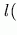v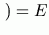v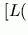X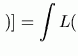X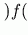x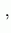v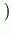dx (120.1)

where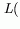X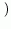defines the system performance and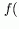x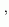vis an (absolutely continuous joint) probability density function in the vector of random variables X.

For system optimization or design refinement, it is helpful to have available the sensitivity ofXto (small) changes in X and hence to (small) changes in the parameters v. WhenXis not explicit, that is, there are no known analytical expressions for (any or all) the performance function(s), Monte Carlo simulation employing crude finite differences is the most common method for sensitivity estimation. It is computationally very demanding.

The approach proposed herein to estimate the gradients and sensitivities at the point of maximum likelihood x* and to construct a 'response surface'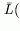Xto approximate the actual performance function. This may then be used to estimate the gradients and sensitivities. Such a surface may be constructed from the some or all of the realizations (samples) from a Monte Carlo analysis used to estimate Equation (120.1):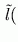vv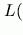X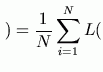X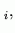v(120.2)

that is, the 'expected' system performance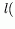vestimated from the average of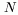system performance evaluations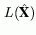. To approximate the actual performance function a tangent hyper-plane is adopted to approximate the original performance function at x*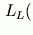x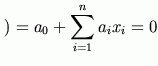(120.3)

where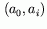,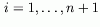are coefficients determined through the fitting of the plane to the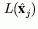.

The gradients (e.g.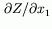, etc.) are obtained from the direction cosines to the hyper-plane obtained from the coefficients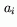. For parameter sensitivities only the mean and standard deviation are considered (e.g.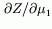etc. and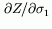etc.). A robust if unsophisticated approach is to use the tangent hyper-plane and finite differences based on the results from the (one) Monte Carlo analysis.

When there are constraint functions G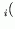x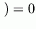,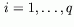on the values x may assume and if x* falls in the inadmissible space Gx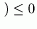,, the formulation is slightly modified. Typically, there will be one or more points x*j,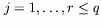but usually only one will be of interest.

One example is given to illustrate that the approximate technique produces results for gradient and for sensitivities very close to the theoretical results. The second example shows how the gradients and sensitivities change with changes in the constraint equation. The change in behaviour is smooth, indicating a gradual change in sensitivity as the boundary moves. However, when the boundary reaches the point of maximum likelihood for the unconstrained performance function the gradients are then uninfluenced by a change in location of the constraint. There are corresponding jump changes in parameter sensitivities. The reason for this is discussed.

The examples illustrate that the proposed technique produces satisfactory results even for highly nonlinear performance functions, implying very considerable approximations in the use of a tangent hyperplane. Importantly, the results for gradients and sensitivities were obtained without repeated Monte Carlo simulation runs. The proposed technique requires little computational effort beyond that required for the original Monte Carlo simulation.

It was found that the proposed technique provided a satisfactory approach for estimating gradients and parameter sensitivities for a number of highly non-linear performance functions, including some with constraints on admissible regions.

References
1
Melchers R.E. and Ahammed M. (2002) Gradient estimation for applied Monte Carlo analyses, Reliability Engineering and System Safety, 78(3) 283-288. doi:10.1016/S0951-8320(02)00172-2

purchase the full-text of this paper (price £20)